# ML Aggarwal Class 8 Solutions for ICSE Maths Chapter 9 Direct and Inverse Variation Check Your Progress

## ML Aggarwal Class 8 Solutions for ICSE Maths Chapter 9 Direct and Inverse Variation Check Your Progress

Question 1.
Match each of the entries in column I with the appropriate entry in column II.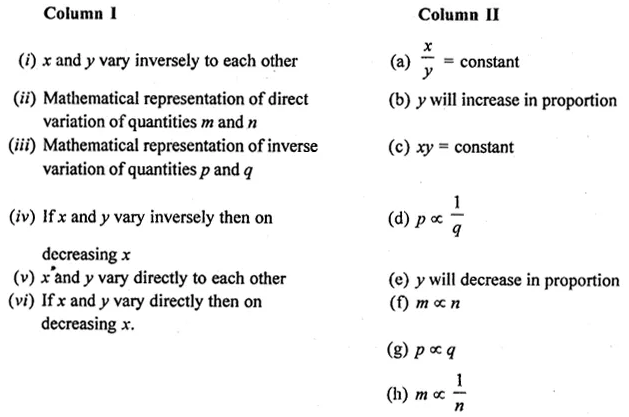Solution: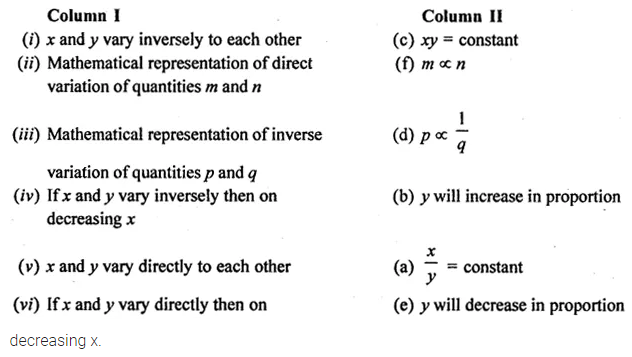Question 2.
(i) From the following table, determines and q if x and y vary directly: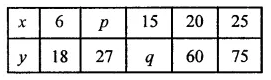(ii) From the following table, determine a and b if x and y vary inversely:Solution: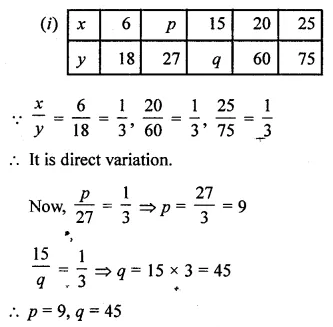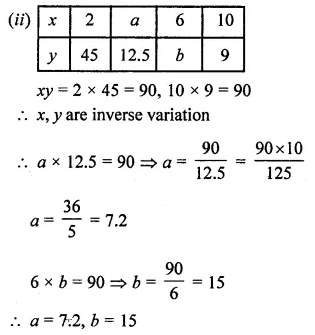Question 3.
It rained 80 mm in the first 20 days of April. What would be the total rainfall in April?
Solution:Question 4.
Mamta earns ₹540 for a working week of 48 hours. If she was absent for 6 hours, how much did she earn?
Solution: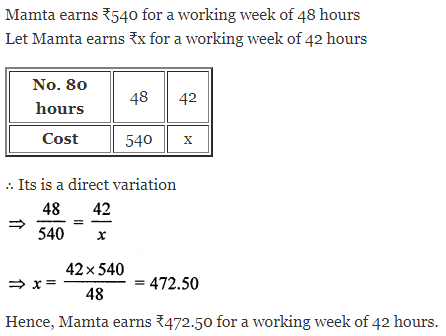Question 5.
Navjot can do a piece of work in 6 days working 10 hours per day. In how many days can he do the same work if he increases his working hours by 2 hours per day?
Solution:Question 6.
Sharmila has enough money to buy 24 bananas at the rate of ₹ 1·50 per banana. How many bananas she can buy if the price of each orange is decreased by 30 paise?
Solution: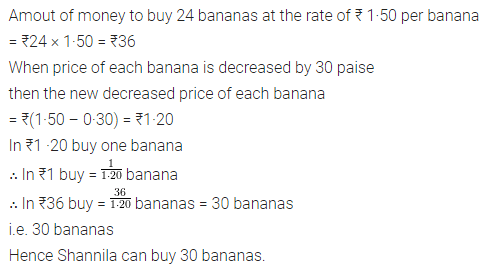Question 7.
A fort has rations for 180 soldiers for 40 days. After 10 days, 30 soldiers leave the fort. Find the total number of days for which the food will last.
Solution: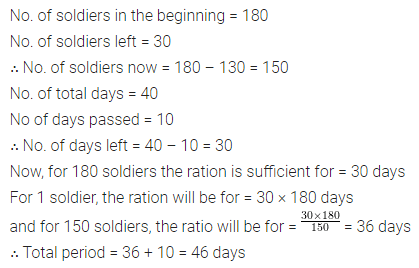Question 8.
There are 100 students in a hostel. Food provision for them is for 20 days. How long will these provisions last, if 25 more students join the group?
Solution: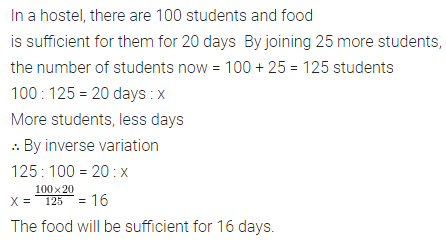Question 9.
If 4 goats or 6 sheep can graze a field in 40 days, how many days will 4 goats and 14 sheep take to graze the same field?
Solution:Question 10.
A tap can fill a tank in 20 hours, while the other can empty it in 30 hours. The tank is empty and if both taps are opened together, how long will it take for the tank to be half full?
Solution: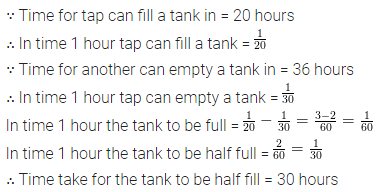Question 11.
Three ants separately can gobble a grasshopper in 3,4, and 6 days respectively. How many days will they take together to finish off the poor chap? If the grasshopper weighs 63 gram, find the share of each.
Solution: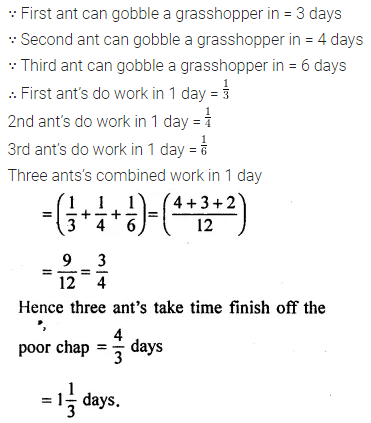Question 12.
A and B together can do a piece of work in 12 days; B and C together can do it in 15 days. If A is twice as good a workman as C, in how many days A alone will do the same work?
Solution: